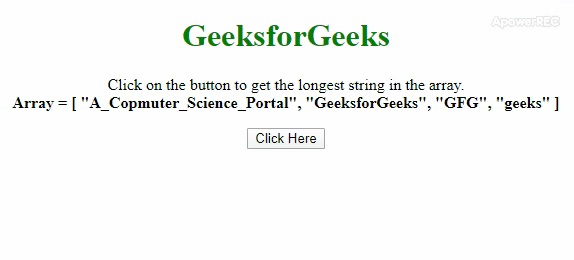Related Articles
How to get the longest string in an array using JavaScript ?
• Last Updated : 31 Dec, 2019

The task is to get the longest string from the array. Here a few of the most used techniques discussed with the help of JavaScript. In this article, we will use two JavaScript methods sort() method and the reduce() method to find out the longest string the array. Both the approach are described below with the example.

Approach 1: In this approach we will use .sort() method which calls a function on every 2 elements of the array. It takes ‘a’ and ‘b’ 2 arguments and compares their length. If the answer is positive then ‘b’ is greater else ‘a’ is greater. This method arranges the elements in the decreasing order of their length and we can access the first element by .

• Example: This example implements the above approach.

 ` ` `<``html``> ` ` `  `<``head``> ` `    ``<``title``> ` `        ``How to get the longest string ` `        ``in an array using JavaScript ? ` `    `` ` `     `  `    ``<``style``> ` `        ``body { ` `            ``text-align: center; ` `        ``} ` `        ``h1 { ` `            ``color: green; ` `        ``} ` `        ``#geeks{ ` `            ``font-weight: bold; ` `        ``} ` `    `` ` ` ` ` `  `<``body``> ` `    ``<``h1``>GeeksforGeeks ` `     `  `    ``<``p``> ` `        ``Click on the button to get the ` `        ``longest string in the array.<``br``> ` `        ``<``b``>Array = [ ` `                ``"A_Copmuter_Science_Portal",  ` `                ``"GeeksforGeeks",  ` `                ``"GFG",  ` `                ``"geeks" ` `            ``] ` `        `` ` `    `` ` `     `  `    ``<``button` `onclick``=``"gfg_Run()"``> ` `        ``Click Here ` `    `` ` `     `  `    ``<``p` `id``=``"geeks"``> ` `     `  `    ``<``script``> ` `        ``var el_down = document.getElementById("geeks"); ` `         `  `        ``var arr = [ ` `            ``"A_Copmuter_Science_Portal",  ` `            ``"GeeksforGeeks", ` `            ``"GFG",  ` `            ``"geeks" ` `        ``]; ` `         `  `        ``// It compares the length of an element with ` `        ``// every other element and after sorting ` `        ``// them in decreasing order it returns the ` `        ``// first element. ` `        ``function gfg_Run() { ` `            ``el_down.innerHTML = "'" +  ` `                    ``arr.sort(function(a, b) { ` `                ``return b.length - a.length; ` `            ``}) + "' is the longest string" ` `                    ``+ " in the array."; ` `        ``} ` `    `` ` ` ` ` `  ` `

• Output:Approach 2: In this approach we will use .reduce() method which calls a function on every 2 elements of the array. It takes ‘a’ and ‘b’ 2 arguments and compares their length. It returns the elements which has its length greater than every element.

• Example: This example implements the above approach.

 ` ` `<``html``> ` ` `  `<``head``> ` `    ``<``title``> ` `        ``How to get the longest string ` `        ``in an array using JavaScript ? ` `    `` ` `     `  `    ``<``style``> ` `        ``body { ` `            ``text-align: center; ` `        ``} ` `        ``h1 { ` `            ``color: green; ` `        ``} ` `        ``#geeks{ ` `            ``font-weight: bold; ` `        ``} ` `    `` ` ` ` ` `  `<``body``> ` `    ``<``h1``>GeeksforGeeks ` `     `  `    ``<``p``> ` `        ``Click on the button to get the ` `        ``longest string in the array.<``br``> ` `        ``<``b``>Array = [ ` `            ``"A_Copmuter_Science_Portal",  ` `            ``"GeeksforGeeks",  ` `            ``"GFG",  ` `            ``"geeks" ` `            ``] ` `        `` ` `    `` ` `     `  `    ``<``button` `onclick``=``"gfg_Run()"``> ` `        ``Click Here ` `    `` ` `     `  `    ``<``p` `id``=``"geeks"``> ` `     `  `    ``<``script``> ` `        ``var el_down = document.getElementById("geeks"); ` `         `  `        ``var arr = [ ` `            ``"A_Copmuter_Science_Portal",  ` `            ``"GeeksforGeeks", ` `            ``"GFG",  ` `            ``"geeks" ` `        ``]; ` `         `  `        ``// It compares the length of a element with ` `        ``// every other element and return it if its ` `        ``// greater than every other element. ` `        ``function gfg_Run() { ` `                ``el_down.innerHTML = "'" +  ` `                ``arr.reduce(function (a, b) {  ` `                    ``return a.length > b.length ? a : b;  ` `                ``}) + "' is the longest string" ` `                    ``+ " in the array.";  ` `            ``} ` `    `` ` ` ` ` `  ` `

• Output:My Personal Notes arrow_drop_up
Recommended Articles
Page :